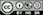Using random sampling to illustrate the Central Limit Theorem

### What is the Central Limit Theorem?

“In probability theory, the central limit theorem (CLT) states that, given certain conditions, the arithmetic mean of a sufficiently large number of iterates of independent random variables, each with a well-defined expected value and well-defined variance, will be approximately normally distributed, regardless of the underlying distribution. That is, suppose that a sample is obtained containing a large number of observations, each observation being randomly generated in a way that does not depend on the values of the other observations, and that the arithmetic average of the observed values is computed. If this procedure is performed many times, the central limit theorem says that the computed values of the average will be distributed according to the normal distribution (commonly known as a ‘bell curve’).”

Source: Wikipedia

## Sample

To generate samples select a distribution from the Distribution dropdown and accept (or change) the default values. Then press Sample or press CTRL-enter (CMD-enter on mac) to run the simulation and show plots of the simulated data.

### R-functions

For an overview of related R-functions used by Radiant for probability calculations see Basics > Probability

© Vincent Nijs (2019)